# Geometry

#### Coordinate Geometry - 2

Mensuration - 1

Mensuration :

Mensuration is the branch of geometry that deals with measuring the length, area and volume of objects. In our earlier lessons, we have already dealt with areas of two dimensional plane objects. So, in this chapter we will entirely concern ourselves with the study of three dimensional objects- their surface areas and volumes. Now, volume represents the space occupied or contained within a shape or a structure.  It represents how much a container of a particular shape will hold- liquid or any dry substance.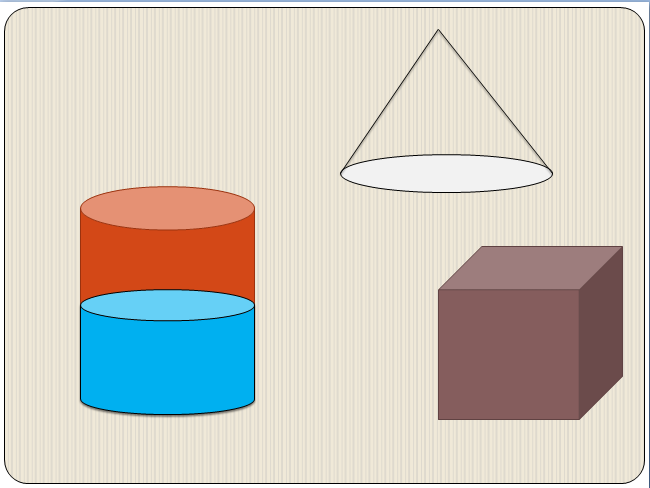One by one we are going to study some of the most standard three dimensional objects and formulae related to their volumes and surface area. We will avoid studying random objects and concern ourselves only with the standard models that frequently appear in exams.

Cube:

A Cube is a three dimensional solid object bounded by fix square faces. A cube has 12 edges, 8 vertices and 6 faces as shown in the figure: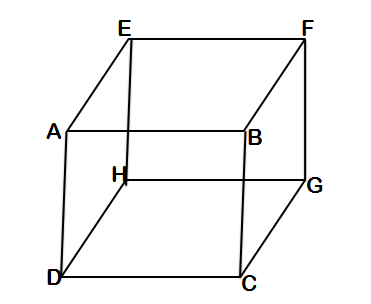Here ABCD, BFGC, EFGH, EHDA, HGCD and EFBA are the six faces while AB, BF, EF, AE, AD, BC, FG, EH, HD, GH, GC and CD are the 12 edges. The following are the properties and formulae related to cubes:Example 1:  If the volumes of two cubes are in the ratio is 8:27. Then what is the ratio of the surface area of the circum spheres of these cubes?

Solution:  If the volumes of the cubes are in the ratio of 8:27, then the side lengths are in the ratio of 2:3. Also, surface area is proportional to the square of the radius of the sphere and the radius of the circum sphere is √3(side)/2 , so

Ratio of surface area of the circum spheres = (2/3)2 = 4:9Example 3 : An ant is sitting at the vertex A and a sugar particle is placed at F. What is the least distance that the ant has to travel to reach the sugar cube if AB = 3 units and the ant travels along the walls of the cube.

Solution: The best way to approach this problem is to visualize a virtual plane that cuts this cube along AD and ignoring the faces ABGH & CDEF, we get,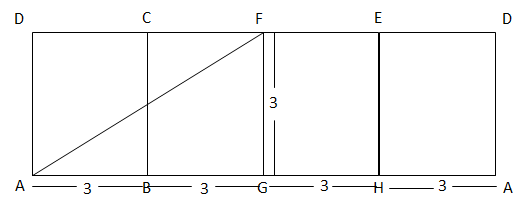Cuboid :

A cuboid is a three dimensional solid formed by six rectangular faces. It also has 8 vertices, 12 edges and 6 faces.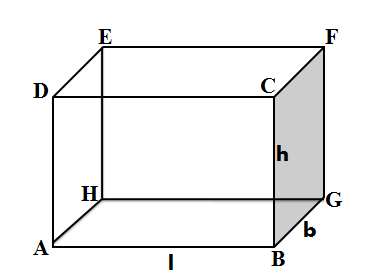Example 4 : The sum of the sides of a cuboid is 112 while the total surface area is 384. What is the radius of the circumsphere circumscribing the cuboid?

Solution: Let the length, breadth and height of the cuboid be l,b and h units

So, 4l + 4b + 4h = 112  or l + b + h = 28 (1)

Also,  2(lb+bh+lh) = 384        (2) Total surface area

Squaring equation (1) we getPyramids

A pyramid is a three dimensional solid formed by joining a polygonal base to an apex point. The base could be a regular polygon or even an irregular one. Each base edge and the apex point form a triangle and is called a lateral face. If a pyramid has n sided base, then there are n+1 vertices, n+1 faces and 2n edges.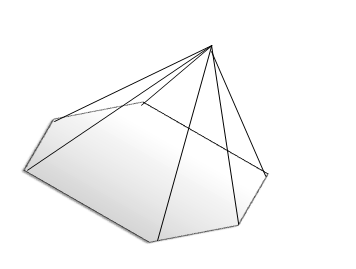A right pyramid means its apex lies directly above the centroid of the base of the pyramid.

Let us consider a regular pyramid with base as square. Each lateral face is a triangle whose height is called the lateral height.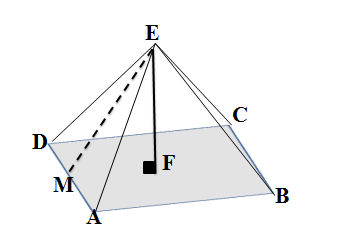Here EM is the lateral height of the face EDA while EF is the height of the pyramid i.e.  the perpendicular distance of the apex point from the base ABCD.

Let the lateral height of each face = lPrism :

Prism is a three dimensional solid with a polygonal base and also a second polygonal base which is exactly the same as the first and parallel to it and the other faces join these two polygonal bases. In a right prism, the joining edges and the faces are perpendicular to each other.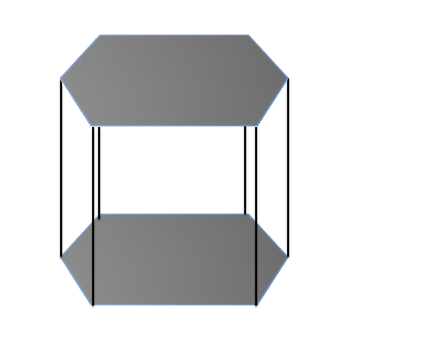Volume of prism = (Area of base) * Height

Example 4: In a right square pyramid, the lateral face are equilateral triangles what is the height of the pyramid if the side length of the pyramid is 4 units.Example  5: The volume of a pyramid with a square base is 200 cm3. The height of the pyramid is 13 cm. What will be the length of the slant edges (i.e. the distance between the apex and any other vertex), rounded to the nearest integer? (XAT 2017)

A. 12 cm         B. 13 cm C. 14 cm         D. 15 cm E. 16 cm

Solution:  Let the square pyramid be ABCDE as shown in the figure

Volume = 1/3 * (Area of base) * height

200 = 1/3 (a2) * 13

a2 = 600/13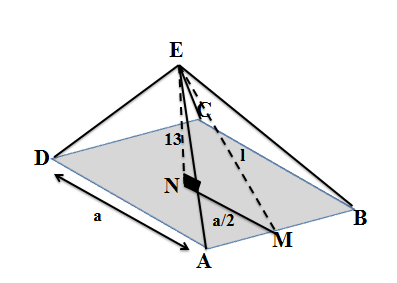Consider ?ENM,

EM2 = EN2 + MN2

l2 = 132 + (a/2)2

l2 = 169 + a2/4

l2 = 169 + 150/13

l2 = 2347/13 =  180.54

Or l = 14 (approximately)

Example 6: In a square pyramid ABCDE with base ABCD and E being the apex point, the area of ?AEB is 140 and area of ?ECD is 160 while that of ABCD is 400 what is the volume of the pyramid?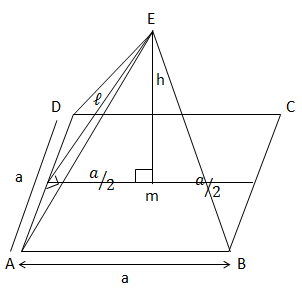Solution: area of ABCD = 400

so AB = BC = CD = AD = 20Cone and Cylinder

Cone

A right circular cone is an example of a pyramid where the base is circle and the axis passes through the centre of the base at right angle to the base. The slant height is usually denoted by l, height of the cone by ‘h’ and the radius of the base as ‘r’.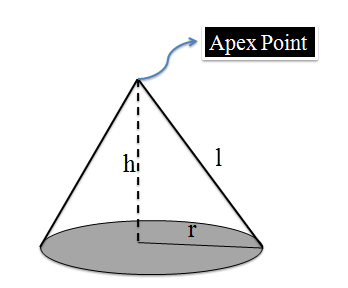Cylinder

It is an example of a prism with a circular base and top.  The circular ends are perpendicular to the axis of the cylinder. It has a fixed height ‘h’ and radius of the base ‘r’.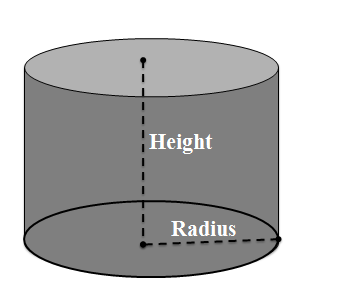Example 7 : A cylinder of maximum volume is placed inside a cube and a cone of maximum volume is placed inside the cylinder. The ratio of their volumes is-

(Take π = 22/7)

A.    A. 20:33:12               B. 33: 20: 11 C. 42:33:11          D. None of these

Solution: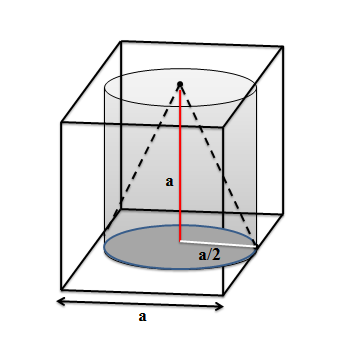As it can be seen from the figure, the height of the cylinder and the cone will be equal to the side length of the cube while the radius of the cylinder and the cone will be equal to half the side length of the cube.Solution: consider ?ADE, AFG and ABC

The three triangles are similar to each other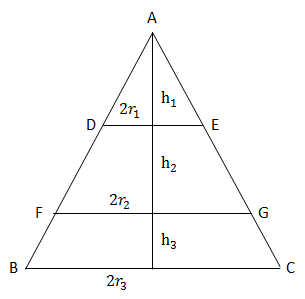So the ratio of their height is the same as ratio of their radii

Also volume r2h.

So ratio of volume of the three cones will be cube of the ratio their heights.

Or heights will be in the ratio of  cube root of their volumesExample 9 : A right angled triangle whose two perpendicular sides are 6 and 8 units, is revolved about its hypotenuse. Find the volume of the double cone so formed.

Solution:Sphere:

A sphere is a perfectly round three dimensional geometrical figure. It resembles a ball in shape. The points on the surface of the surface of the sphere are all at a fixed distance from the centre.